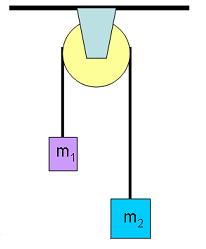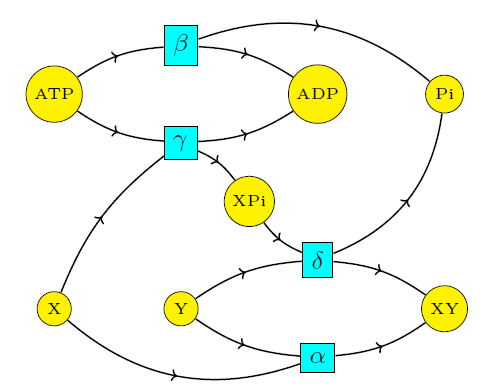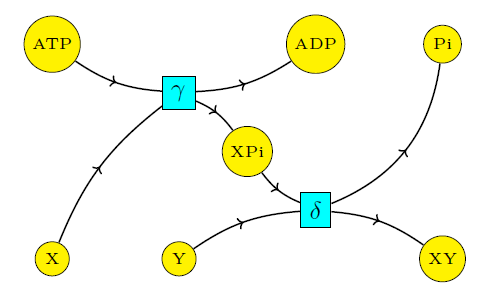## Coupling Through Emergent Conservation Laws (Part 6)

#### John Baez, Jonathan Lorand, Blake Pollard, and Maru Sarazola

Now let's think about emergent conservation laws!

When a heavy rock connected to a lighter one by a pulley falls down and pulls up the lighter one, you're seeing an emergent conservation law:Here the height of the heavy rock plus the height of light one is a constant. That's a conservation law! It forces some of the potential energy lost by one rock to be transferred to the other. But it's not a fundamental conservation law, built into the fabric of physics. It's an emergent law that holds only thanks to the clever design of the pulley. If the rope broke, this law would be broken too.

It's not surprising that biology uses similar tricks. But let's see exactly how it works. First let's look at all four reactions we've been studying:$$\begin{array}{cccc} \mathrm{X} + \mathrm{Y} & \mathrel{\substack{\alpha_{\rightarrow} \\\longleftrightarrow\\ \alpha_{\leftarrow}}} & \mathrm{XY} & \qquad (1) \\ \\ \mathrm{ATP} & \mathrel{\substack{\beta_{\rightarrow} \\\longleftrightarrow\\ \beta_{\leftarrow}}} & \mathrm{ADP} + \mathrm{P}_{\mathrm{i}} & \qquad (2) \\ \\ \mathrm{X} + \mathrm{ATP} & \mathrel{\substack{\gamma_{\rightarrow} \\\longleftrightarrow\\ \gamma_{\leftarrow}}} & \mathrm{ADP} + \mathrm{XP}_{\mathrm{i}} & \qquad (3) \\ \\ \mathrm{XP}_{\mathrm{i}} +\mathrm{Y} & \mathrel{\substack{\delta_{\rightarrow} \\\longleftrightarrow\\ \delta_{\leftarrow}}} & \mathrm{XY} + \mathrm{P}_{\mathrm{i}} & \qquad (4) \end{array}$$

It's easy to check that the rate equations for these reactions have the following conserved quantities, that is, quantities that are constant in time:

A)  $$[\mathrm{X}] + [\mathrm{XP}_{\mathrm{i}} ] + [\mathrm{XY}],$$ due to the conservation of X.

B)  $$[\mathrm{Y}] + [\mathrm{XY}],$$ due to the conservation of Y.

C)  $$3[\mathrm{ATP}] +[\mathrm{XP}_{\mathrm{i}} ] +[\mathrm{P}_{\mathrm{i}}] +2[\mathrm{ADP}],$$ due to the conservation of phosphorus.

D)  $$[\mathrm{ATP}] + [\mathrm{ADP}],$$ due to the conservation of adenosine.

Moreover, these quantities, and their linear combinations, are the only conserved quantities for reactions (1)–(4).

To see this, we use some standard ideas from reaction network theory. Consider the 7-dimensional space with orthonormal basis given by the species in our reaction network:

$$\mathrm{ATP}, \mathrm{ADP}, \mathrm{P}_{\mathrm{i}}, \mathrm{XP}_{\mathrm{i}}, \mathrm{X}, \mathrm{Y}, \mathrm{XY}$$

We can think of complexes like $$\mathrm{ADP} + \mathrm{P}_{\mathrm{i}}$$ as vectors in this space. An arbitrary choice of the concentrations of all species also defines a vector in this space. Furthermore, any reaction involving these species defines a vector in this space, namely the sum of the products minus the sum of the reactants. This is called the reaction vector of this reaction. Reactions (1)–(4) give these reaction vectors:

$$\begin{array}{ccl} v_\alpha &=& \mathrm{XY} - \mathrm{X} - \mathrm{Y} \\ \\ v_\beta &= & \mathrm{P}_{\mathrm{i}} + \mathrm{ADP} - \mathrm{ATP} \\ \\ v_\gamma &=& \mathrm{XP}_{\mathrm{i}} + \mathrm{ADP} - \mathrm{ATP} - \mathrm{X} \\ \\ v_\delta &= & \mathrm{XY} + \mathrm{P}_{\mathrm{i}} - \mathrm{XP}_{\mathrm{i}} - \mathrm{Y} \end{array}$$

Any change in concentrations caused by these reactions must lie in the stoichiometric subspace: that is, the space spanned by the reaction vectors. Since these vectors obey one nontrivial relation:

$$v_\alpha + v_\beta = v_\gamma + v_\delta$$

the stochiometric subspace is 3-dimensional. Therefore, the space of conserved quantities must be 4-dimensional, since these specify the constraints on allowed changes in concentrations.

Now let's compare the situation where 'coupling' occurs! For this we consider only reactions (3) and (4):Now the stoichiometric subspace is 2-dimensional, since $$v_\gamma$$ and $$v_\delta$$ are linearly independent. Thus, the space of conserved quantities becomes 5-dimensional! Indeed, we can find an additional conserved quantity:

E)  $$[\mathrm{Y} ] +[\mathrm{P}_{\mathrm{i}}]$$

that is linearly independent from the four conserved quantities we had before. It does not derive from the conservation of a particular molecular component. In other words, conservation of this quantity is not a fundamental law of chemistry. Instead, it is an emergent conservation law, which holds thanks to the workings of the cell! It holds in situations where the rate constants of reactions catalyzed by the cell's enzymes are so much larger than those of other reactions that we can ignore those other reactions.

And remember from last time: these are precisely the situations where we have coupling.

Indeed, the emergent conserved quantity E) precisely captures the phenomenon of coupling! The only way for ATP to form ADP + Pi without changing this quantity is for Y to be consumed in the same amount as Pi is created... thus forming the desired product XY.

Next time we'll look at a more complicated example from biology: the urea cycle.